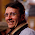Monday, December 5, 2011

Generate all the subsets of a given set

This could be tricky unless you realize that given an set of size n there are 2^n subsets. An element can be in or out, so 0/1 and this means that you just need to generate all the binary numbers from 0  to n. The binary representation of each number will tell you whether or not you need to include the element (bit i = 1 means element in).

1.T[] arr = ...;
var subsets = from m in Enumerable.Range(0, 1 << arr.Length) select from i in Enumerable.Range(0, arr.Length) where (m & (1 << i)) != 0 select arr[i];

2.This comment has been removed by the author.

3.My solution using backtracking

http://www.geeksforgeeks.org/archives/14469

4.There is another one which is better and simpler